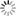# Episode List

OR

### 1952

1 Jan. 1952
Love TrapBe the first one to add a plot.

8 Jan. 1952
Fresh as a DaisyBe the first one to add a plot.

15 Jan. 1952
The IntrudersBe the first one to add a plot.

22 Jan. 1952Be the first one to add a plot.

29 Jan. 1952
WindfallBe the first one to add a plot.

5 Feb. 1952
Prelude to DeathBe the first one to add a plot.

12 Feb. 1952
The Hands of the EnemyBe the first one to add a plot.

19 Feb. 1952
Primary DecisionBe the first one to add a plot.

4 Mar. 1952
Benefit PerformanceBe the first one to add a plot.

18 Mar. 1952
The Way of FreedomBe the first one to add a plot.

25 Mar. 1952
The HeroBe the first one to add a plot.

1 Apr. 1952
The Escape ArtistBe the first one to add a plot.

8 Apr. 1952
The Black TieBe the first one to add a plot.

15 Apr. 1952
Cornbelt KidBe the first one to add a plot.

22 Apr. 1952
Hello, Mr. LutzBe the first one to add a plot.

29 Apr. 1952
Border IncidentBe the first one to add a plot.

6 May 1952
Dark as NightBe the first one to add a plot.

13 May 1952
The Strong FinishBe the first one to add a plot.

20 May 1952
A Buck Is a BuckBe the first one to add a plot.

27 May 1952
The Gold WatchBe the first one to add a plot.

3 Jul. 1952
The Paper Box KidBe the first one to add a plot.

10 Jun. 1952
The SystemA garage mechanic, desperate for money, tries his luck at a shabby, gangster-owned gambling den with what he thinks is a sure formula for predicting dice throws.

17 Jun. 1952
Death Among the RelicsA street-wise kid who begs in a museum sneaks into the building and meets a man who identifies himself as a Secret Service Agent. In fact, the older man is a thief who needs the boy's help to escape when his exit plan is scuppered.

24 Jun. 1952
Death for the LonelyBe the first one to add a plot.

1 Jul. 1952
The Double DealBe the first one to add a plot.

15 Jul. 1952
The Net Draws TightBe the first one to add a plot.

29 Jul. 1952
A Date at MidnightBe the first one to add a plot.

5 Aug. 1952
Murder Takes the 'A' TrainBe the first one to add a plot.

12 Aug. 1952
Flowers of DeathBe the first one to add a plot.

26 Aug. 1952
The Face of FearBe the first one to add a plot.

16 Sep. 1952
Death Signs an AutographBe the first one to add a plot.

30 Sep. 1952
Change UpBe the first one to add a plot.

7 Oct. 1952Be the first one to add a plot.

14 Oct. 1952
ButtonsBe the first one to add a plot.

28 Oct. 1952
Club DateBe the first one to add a plot.

11 Nov. 1952
The FixBe the first one to add a plot.

18 Nov. 1952
The Hanging JudgeBe the first one to add a plot.

25 Nov. 1952
BoomerangBe the first one to add a plot.

2 Dec. 1952
King of the ConsBe the first one to add a plot.

9 Dec. 1952
The GunmanBe the first one to add a plot.

16 Dec. 1952
The Next Stop, DeathBe the first one to add a plot.

30 Dec. 1952
Death Pulls the Strings